# THERMODYNAMICS

Thermodynamics is the study of energy flows in and through systems. The laws of thermodynamics control everything that happens in our universe. Before we look at the laws of thermodynamics, we should consider the concept of a system.

## Systems

The idea of a systems approach to studying the world around us is to treat the "system" as a black box. That is to treat is as something we can not look into, but we can see how it affects its surrounding environment. It is a very effective way to simplify very complex problems.

The properties which define a system are:

• A system has boundaries. These boundaries may be well defined by natural interfaces between the system and its imediate environment, or they may be defined purely for convenience.
• Any quantities which crosses the boundary of a system to enter it is called an input
• Any quantity which criosses the boundary of a system to leave it is called an output
• All systems undergo processes which convert inputs to outputs.

A system can be as simple as a cup of hot water, or as complex as the human body. Systems may have other systems within them. For example:

The system defined by the human body has many other systems within it, such as the circulatory system of heart and blood vessels. Each of these sub-systems have their own boundaries, inputs, outputs and processes. We can look at this process in reverse also. All systems around us can be redefined as sub-systems of larger systems. For example:

The system defined as the human body is a sub-system of the ecosystem of the earth. The ecosystem is a sub-system of the earth itself. The earth is a sub-system of the solar-system. The solar-system is a sub- system of the Milky Way galexy, and so on until we define the system which encapsulates all other systems called the "Universe". So by studying the laws of thermodynamics we are in fact looking at some of the most fundamental properties of the universe itself.

Every system exists in some state. States may change as the system processes inputs, or reacts to its immediate environment. For example:

• Matter can exist in a solid state, a liquid state or a gas state.
• Electrons can be in a low orbital state, a high orbital state (if a quantum of energy such as a photon is applied) or they can be free of any nucli.
• Humans can be in a conscious state or an unconscious state.

There are certain properties of the system which can be measured, which will indicate the state of the system. These properties are known as state variables. To be a state variable, a property must:

1. Be measurable
2. Indicate the state of the system.

Some state variables of the human body are:

• Pulse
• Temperature
• Respiration Rate

These, when measured, can give a very clear indication of the state of the system

All systems are controlled by the laws of thermodynamics:

## THE FIRST LAW

Countless observations have shown that it is impossible to create a system which will produce more energy outputs than the sum of the energy put into it and the energy already contained within the system itself. These observations gave rise to the First Law of Thermodynamics.

Definition: The net input of energy to a system is equal to the net rise in internal energy plus the net output of energy from the system.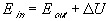### Implications of the First Law

• Energy can neither be created nor destroyed. This is known as "Conservation of Energy"
• The total energy of an isolated system is fixed.
• As the universe is an isolated system, the total energy of the universe is fixed. The universe came into being with a fixed amount of energy. There is no mechanism for creating more energy.

It should be noted that these implications apply equally to matter as well as energy. As Einstine showed earlier this century, energy and matter are essentially the same thing. In fact the energy contained in matter is given by his famous equation:

 E = mc2 where E = the energy equivalent of the matter m = the mass of the matter c = the speed of light

So, a more complete definition of the first law of thermodynamics would be:

The net input of energy/matter to a system is equal to the net rise in internal energy/matter plus the net output of energy/matter from the system

### Enthalpy

Enthalpy is a measure of the total internal energy of a system. It is a combination of both kinetic and potential energy.

## THE SECOND LAW

Countless observations of systems have shown that when a system is not in equilibrium either with its surroundings or internally, it undergoes spontaneous transfer of energy until it reaches its lowest equilibrium state. This can be seen when you have a hot object in cooler surroundings. There is a spontaneous transfer of heat from the object to its surroundings until their temperatures equalise.

An important point to notice about these spontaneous transfers of energy is that they are irreversible. This implies that the energy transfered can not be recovered by the system. The energy is no longer available.

This is as a result of the second law of thermodynamics.

Definition: Among all the allowable states of a system with a given amount of energy, one and only one is a stable equilibrium state. If the system is not at this stable equilibrium state it will spontaneously change state until it reaches equalibrium.

### Implications of the Second Law

• By virtue of the second law, stable states must exist for every system
• By virtue of the second law, irreversible processes must take place
• By virtue of the second law, if a system is undergoing irreversible processes it can not have reached its stable equilibrium state.
• We observe many irreversible processes taking place around us in the universe, therefore the universe has not yet reached its stable equilibrium state.

### Entropy

To fully appreciate the concept of entropy, it is necessary to digress slightly into the realm of statistics.

Consider a deck of cards, arranged in order and thrown into the air. It is more probable for the cards to fall in random order and arrangement, than for them to fall in order. We expect this because there are many more options for the cards to fall in a disordered way, but only one way for the cards to fall in order.

The same laws of probability apply to the ordering within distributions of particles in systems. Consider, for example, the melting of an ice cube. Of all the possible ways of placing water molicules into a container, the highly ordered arrangement within the crystalline ice cube has a very low probability, while the disordered arrangement of the molicules in a liquid is more probable.

Because statistical probability is so important in determining the outcome of spontaneous events, thermodynamics defines a quantity, called entropy (S), that describes the degree of randomness of a system. The larger the value of entropy, the more random (disordered) the system is.

Because all systems undergo irreversible processes, it is implied that energy is no longer freely available to the system for conversion. The quantity, Entropy is also a measure of this unavailable” energy.

## Temperature

### What is Temperature?

In a qualitative manner, we can describe the temperature of an object as that which determines the sensation of warmth or coldness felt from contact with it.

It is easy to demonstrate that when two objects are placed together (physicists say when they are put in thermal contact), the hotter object cools while the cooler object becomes warmer until a point is reached after which no more change occurs, and to our senses, they feel the same degree of warmth or coolness. When the thermal changes have stopped, we say that the two objects (physicists define them more rigorously as systems) are in thermal equilibrium . We can then define the temperature of the system by saying that the temperature is that quantity which is the same for both systems when they are in thermal equilibrium.

If we experiment further with more than two systems, we find that many systems can be brought into thermal equilibrium with each other; thermal equilibrium does not depend on the kind of object used. Put more precisely,

 if two systems are separately in thermal equilibrium with a third, then they must also be in thermal equilibrium with each other,

and they all have the same temperature regardless of the kind of systems they are.

The statement in italics, called the zeroth law of thermodynamics may be restated as follows:

 If three or more systems are in thermal contact with each other and all in equilibrium together, then any two taken separately are in equilibrium with one another. (quote from T. J. Quinn's monograph Temperature)

Now one of the three systems could be an instrument calibrated to measure the temperature - i.e. a thermometer. When a calibrated thermometer is put in thermal contact with a system and reaches thermal equilibrium, we then have a quantitative measure of the temperature of the system. For example, a mercury-in-glass clinical thermometer is put under the tongue of a patient and allowed to reach thermal equilibrium in the patient's mouth - we then see by how much the silvery mercury has expanded in the stem and read the scale of the thermometer to find the patient's temperature.

Temperature is the potential which governs the flow of heat into and out of systems .

### Absolute Temperature

It would be useful to define an absolute scale of temperature.

Experiments with gases have shown there is a very simple relation between their pressure, volume, and temperature:

 pV= (constant)T.

This temperature is called the thermodynamic temperature and is now accepted as the fundamental measure of temperature. Note that there is a naturally-defined zero on this scale - it is the point at which the pressure of an ideal gas is zero, making the temperature also zero. With this as one point on the scale, only one other fixed point need be defined. In 1933, the International Committee of Weights and Measures adopted this fixed point as the triple point of water , the temperature at which water, ice, and water vapor coexist in equilibrium); its value is set as 273.16. The unit of temperature on this scale is called the kelvin, after Lord Kelvin (William Thompson), 1824-1907, and its symbol is K (no degree symbol used).

To convert from Celsius to Kelvin, add 273.16.

K = ° C + 273.16. (Note: This is often simplified to: K = ° C + 273)

Thermodynamic temperature is the fundamental temperature; its unit is the kelvin which is defined as the fraction 1/273.16 of the thermodynamic temperature of the triple point of water.

When measuring temperature the divisions used in the Kelvin scale are equal to the divisions used in the Celsius scale. The only difference is that 0oC = 273.16o K

Thus:

oC oK
0 273.16
1 274.16
2 275.16
10 283.16
100 373.16

As energy flow is related to Entropy, a suitable way of defining an “absolute” scale of temperature must include both energy and entropy. Thus: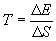where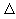E = change in energyS = change in entropy T = temperature in oK

### Exercise

Consider two systems A & B in thermal contact, but at different temperatures. Experience shows that heat will flow from the higher temperature to the lower temperature until the temperatures equalise.

Thus the change in energy of system A will be -Q, the negative sign indicating a loss of internal energy from the system. The change in energy of system B will be Q by virtue of the first law.

 Thus for system A: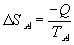and for system B: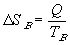If we now redefine the system of interest to consider the larger system which includes both system A and B then: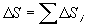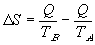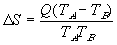Since TA > TB ,S is always > 0. Ie. The entropy of the larger system will always increase.

### Some Implications of this Exercise

• When a system undergoes irreversible processes the entropy of the system will always increase.
• Entropy for a system is at a maximum only when the system reaches its stable equalibrium state.
• If entropy is increasing, the system is progressing from an ordered state to a disordered state.
• For a system to become ordered, requires controlled input from outside that system ! Thus to establish and maintain a highly ordered system such as a living creature (or even an entire ecosystem) requires controlled inputs of energy. This is why plants need sun light to manufacture food, and animals need to constantly eat to maintain their life.
• As we showed earlier, the Universe is a system which encloses all possible sub systems. We know from observation that these sub-systems are undergoing irreversible processes. Therefore the entropy of the universe is always increasing.
• If the universe is infinite in size, then the entropy of the universe will head toward infinity. This means that the universe is headed toward infinite disorder. Thus
• Knowing which direction this process is headed, if we look backward in time, the universe must once have been more highly ordered than it is now.
• The universe is winding down !!

Now the prudent observer of this information will see immediately that there is a fundamental problem.